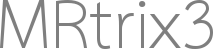# 5ttgen output act.mif with only zeros inside

Hi,

I am running 5ttgen on a T1_2_diff.nii.gz (T1 in diffusion space).
5ttgen does not fail, but the output only contains 0s, both with fsl and freesurfer options.
Specifically, I run:
5ttgen fsl T1_2_diff.nii.gz act.mif
I also tried directly on the T1.nii.gz, with the same result.
The T1 looks normal (see bleow)

Any ideas?

T1:

act.mif:

Tim

Hi Tim,

I’ve never seen `5ttgen` give a completely blank image. So my only advice is to run the script with the `-nocleanup` option. The temporary directory will include the file `log.txt`, which will tell you exactly what commands were executed and in which order. You can cross-reference this with the intermediate files within the temporary directory to find the first command at which the results are not as expected given the input.

Note also that the `5ttgen freesurfer` algorithm requires a different type of image input (a parcellation image) to `5ttgen fsl` (a T1-weighted image). Feeding `5ttgen freesurfer` a T1-weighted image and getting a blank output image would be unsurprising.

Rob

Hi Rob,

thanks for the answer.I believe it is `fast T1_BET.nii.gz` that fails, giving me empty files (‘0’ in the middle (maybe range of the brain?) and ‘?’ outside) for T1_BET_pve_1.nii.gz and T1_BET_pve_2.nii.gz. T1_BET.nii.gz seems normal, see below. Any clues?

T1_BET.nii.gz

T1_BET_pve_1.nii.gz ‘0’ values

T1_BET_pve_1.nii.gz, '?" values

For 5ttgen with Freesurfer, thanks, I did not realize that, it is working correctly with the aparc+aseg.mgz.

Cheers, Tim

It would be worthwhile confirming this by running `fast` directly on the image `T1_BET.nii.gz`, outside of the MRtrix3 scripts, in order to confirm that the issue is indeed arising from that command and not due to MRtrix3’s wrapping of it. If that is indeed the case, there’s a couple of things you can try:

• Run `mrstats` on `T1_BET.nii.gz`, just to see if there’s any particular unusual values in the image (in particular, make sure that the “count” field does indeed correspond to the number of voxels in the image expected given the image dimensions).

• Run `fast` with the `--verbose` option, and see if it gives any meaningful warning or error messages.

The output I provided in my last messages where actually for `fast T1_BET.nii.gz` that I ran outside of mrtrix. mrstats gives consistent results (the count field is as expected).
Here is the output of `fast --verbose T1_BET.nii.gz` (I see some nans that look suspicious):

``````T1-weighted image
Imagesize : 181 x 238 x 208
Pixelsize : 1 x 1 x 1

1 4.11087
2 4.40672
3 4.64439
KMeans Iteration 0
KMeans Iteration 1
KMeans Iteration 2
KMeans Iteration 3
KMeans Iteration 4
KMeans Iteration 5
KMeans Iteration 6
KMeans Iteration 7
KMeans Iteration 8
KMeans Iteration 9
KMeans Iteration 10
KMeans Iteration 11
KMeans Iteration 12
KMeans Iteration 13
KMeans Iteration 14
KMeans Iteration 15
KMeans Iteration 16
KMeans Iteration 17
KMeans Iteration 18
Tanaka Iteration 0 bias field 4
Tanaka-inner-loop-iteration=0 MRFWeightsTotal=1.89965e+07 beta=0.02
Tanaka-inner-loop-iteration=1 MRFWeightsTotal=1.91803e+07 beta=0.02
Tanaka-inner-loop-iteration=2 MRFWeightsTotal=1.91855e+07 beta=0.02
Tanaka-inner-loop-iteration=3 MRFWeightsTotal=1.91857e+07 beta=0.02
Tanaka-inner-loop-iteration=4 MRFWeightsTotal=1.91857e+07 beta=0.02
CLASS 1 MEAN 48.1445 STDDEV 28.0758 CLASS 2 MEAN 83.8878 STDDEV 12.6211 CLASS 3 MEAN 110.759 STDDEV 0.970947
Tanaka Iteration 1 bias field 4
Tanaka-inner-loop-iteration=0 MRFWeightsTotal=1.90828e+07 beta=0.02
Tanaka-inner-loop-iteration=1 MRFWeightsTotal=1.92659e+07 beta=0.02
Tanaka-inner-loop-iteration=2 MRFWeightsTotal=1.92711e+07 beta=0.02
Tanaka-inner-loop-iteration=3 MRFWeightsTotal=1.92713e+07 beta=0.02
Tanaka-inner-loop-iteration=4 MRFWeightsTotal=1.92713e+07 beta=0.02
CLASS 1 MEAN 47.6439 STDDEV 27.6441 CLASS 2 MEAN 84.3282 STDDEV 12.7136 CLASS 3 MEAN 110.712 STDDEV 0.701077
Tanaka Iteration 2 bias field 4
Tanaka-inner-loop-iteration=0 MRFWeightsTotal=1.91363e+07 beta=0.02
Tanaka-inner-loop-iteration=1 MRFWeightsTotal=1.93199e+07 beta=0.02
Tanaka-inner-loop-iteration=2 MRFWeightsTotal=1.93253e+07 beta=0.02
Tanaka-inner-loop-iteration=3 MRFWeightsTotal=1.93255e+07 beta=0.02
Tanaka-inner-loop-iteration=4 MRFWeightsTotal=1.93255e+07 beta=0.02
CLASS 1 MEAN 47.6608 STDDEV 27.6317 CLASS 2 MEAN 84.754 STDDEV 12.8979 CLASS 3 MEAN 110.815 STDDEV 0.488884
Tanaka Iteration 3 bias field 4
Tanaka-inner-loop-iteration=0 MRFWeightsTotal=1.92219e+07 beta=0.02
Tanaka-inner-loop-iteration=1 MRFWeightsTotal=1.9409e+07 beta=0.02
Tanaka-inner-loop-iteration=2 MRFWeightsTotal=1.94147e+07 beta=0.02
Tanaka-inner-loop-iteration=3 MRFWeightsTotal=1.94149e+07 beta=0.02
Tanaka-inner-loop-iteration=4 MRFWeightsTotal=1.94149e+07 beta=0.02
CLASS 1 MEAN 47.8091 STDDEV 27.7284 CLASS 2 MEAN 85.1691 STDDEV 13.1122 CLASS 3 MEAN 110.925 STDDEV 0.243782
Tanaka Iteration 0 hyperparameter 4
Tanaka-inner-loop-iteration=0 MRFWeightsTotal=0 beta=0.02
Tanaka-inner-loop-iteration=1 MRFWeightsTotal=0 beta=0.02
Tanaka-inner-loop-iteration=2 MRFWeightsTotal=0 beta=0.02
Tanaka-inner-loop-iteration=3 MRFWeightsTotal=0 beta=0.02
Tanaka-inner-loop-iteration=4 MRFWeightsTotal=0 beta=0.02
CLASS 1 MEAN 48.0753 STDDEV 27.9426 CLASS 2 MEAN 85.6899 STDDEV 13.4117 CLASS 3 MEAN 110.981 STDDEV -nan
BETA 0.1
Tanaka Iteration 1 hyperparameter 4
Tanaka-inner-loop-iteration=0 MRFWeightsTotal=0 beta=0.1
Tanaka-inner-loop-iteration=1 MRFWeightsTotal=0 beta=0.1
Tanaka-inner-loop-iteration=2 MRFWeightsTotal=0 beta=0.1
Tanaka-inner-loop-iteration=3 MRFWeightsTotal=0 beta=0.1
Tanaka-inner-loop-iteration=4 MRFWeightsTotal=0 beta=0.1
CLASS 1 MEAN -nan STDDEV -nan CLASS 2 MEAN -nan STDDEV -nan CLASS 3 MEAN -nan STDDEV -nan
BETA 0.1
Tanaka Iteration 2 hyperparameter 4
Tanaka-inner-loop-iteration=0 MRFWeightsTotal=0 beta=0.1
Tanaka-inner-loop-iteration=1 MRFWeightsTotal=0 beta=0.1
Tanaka-inner-loop-iteration=2 MRFWeightsTotal=0 beta=0.1
Tanaka-inner-loop-iteration=3 MRFWeightsTotal=0 beta=0.1
Tanaka-inner-loop-iteration=4 MRFWeightsTotal=0 beta=0.1
CLASS 1 MEAN -nan STDDEV -nan CLASS 2 MEAN -nan STDDEV -nan CLASS 3 MEAN -nan STDDEV -nan
BETA 0.1
Tanaka Iteration 3 hyperparameter 4
Tanaka-inner-loop-iteration=0 MRFWeightsTotal=0 beta=0.1
Tanaka-inner-loop-iteration=1 MRFWeightsTotal=0 beta=0.1
Tanaka-inner-loop-iteration=2 MRFWeightsTotal=0 beta=0.1
Tanaka-inner-loop-iteration=3 MRFWeightsTotal=0 beta=0.1
Tanaka-inner-loop-iteration=4 MRFWeightsTotal=0 beta=0.1
CLASS 1 MEAN -nan STDDEV -nan CLASS 2 MEAN -nan STDDEV -nan CLASS 3 MEAN -nan STDDEV -nan
BETA 0.1
Starting Partial Volume Estimation

tissue 1 1.86982e+06
tissue 2 0
tissue 3 0
total tissue 1.86982e+06
``````

Thanks!

`CLASS 1 MEAN 48.0753 STDDEV 27.9426 CLASS 2 MEAN 85.6899 STDDEV 13.4117 CLASS 3 MEAN 110.981 STDDEV -nan`

You could probably interrogate this further by reducing the number of iterations and hence get a look at the intermediate images. My guess looking at the output is that the WM intensities are being enveloped into the Gaussians that represent the GM intensities, eventually resulting in no assignment of voxels to WM and hence a non-finite standard deviation of that distribution. Does your image have particularly poor GM-WM contrast?

I can’t really provide guidance over and above that though: Ultimately it’s an FSL command that’s encountering an issue entirely independently of MRtrix3.

1 Like

Thanks a lot, reducing the number of iteration made it work. I still don’t know why it did not work with the default parameters (the contrast does not seem worse than usual to me), but I will go with that. I realized it is not about MRtrix anymore, so double thanks for you help!